# Numbers and Shapes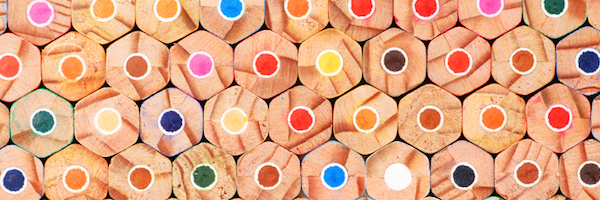You probably know something about Triangular Numbers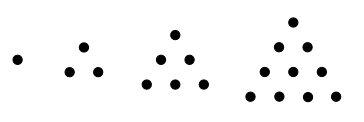etc.

You’re also likely to have come across Square Numbers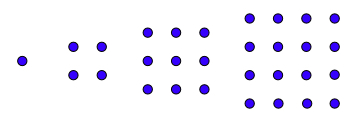etc.

Maybe you’ve explored things like:- 1 + 2 + 3 or 3 + 5 + 7 + 9 or 2 + 4 + 6 + 8 + 10 and 5 + 10 + 15 etc.

The challenge is to use number patterns and look at 'shape numbers'.

Here are the rules, in order:

Rule A/ Use starting numbers 1 or 2 or 3 or 4 or 5 or 6. So choose one of them now. (e.g. 4)

Rule B/ Go in steps of 1 or 2 or 3 or 4 or 5 or 6 and choose one of these steps. (e.g. 3 so:- 4, 7, 10, 13 etc.)

Rule C/ Decide how many numbers to use in the set (three or four or five) (e.g. for three: 4, 7, 10)

Rule D/ Add the sequence 4 + 7 + 10 = 21

Rule E/ Look at sequences of triangular, square, pentagonal and hexagonal numbers and see if there’s a match.

YES! 21 is the 6th triangular number.

See what other triangular, square, pentagonal and hexagonal numbers you find.

Here are the first four pentagonal and hexagonal numbers to help you find many more!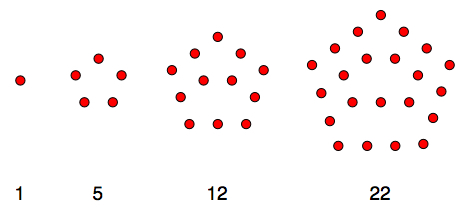etc.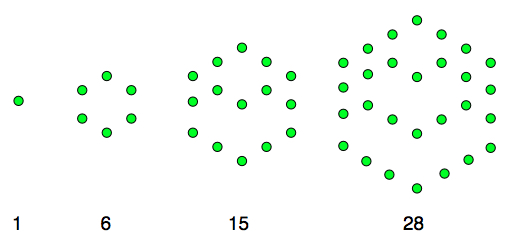etc.

If you want some help starting here are some examples;

A/ Using starting number 3

Going in steps of 2

Using 5 numbers in the set 3 + 5 + 7 + 9 + 11 = 35 the 5th Pentagonal Number

B/ Using starting number 6

Going in steps of 6

Using 3 numbers in the set 6 + 12 + 18 = 36 the 8th Triangular & 6th Square Numbers